马达用词解释

同步转速
• 马达的频率丶极数可以决定马达的转速。参考公式如下：
• Ns = ( 120 x f ) / P
• Ns：同步转速 (rpm)
• 120：常数
• f：频率
• P：极数

无载转速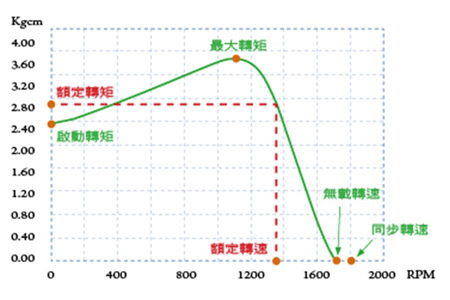输出功率
• 表示马达在一个单位时间内所能做的功，马达所做的功是由转速及转矩来决定。参考公式如下：
• 输出 (Kw) = ( T x N ) / 97400
• T：转矩 (Kgcm)
• N：转速
• 1HP：0.746Kw

转差率
• 属转速表示方法之一，参考公式如下：
• S = ( Ns - N ) / Ns
• S：转差率
• Ns：同步转速 (rpm)
• N：任意负载下的转速 (rpm)

马达选择方法

01
减速比计算公式

• i = Nm / Ng or 1 / i = Ng / Nm
• i：减速比
• Ng：减速机转速 (rpm)
• Nm：马达转速 (rpm)

02
直接连结减速机的转矩计算公式

• Tg = Tm x i x η
• Tg：减速机输出转矩
• Tm：马达输出转矩
• i：减速比
• η：减速机传动效率
最大容许转矩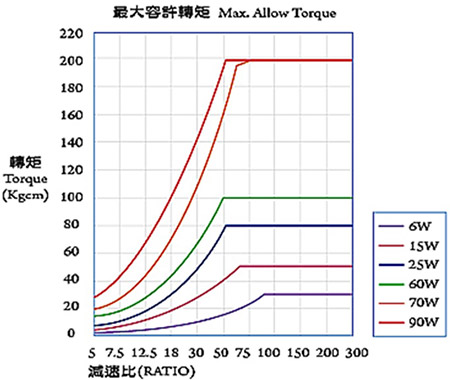马达容量基本公式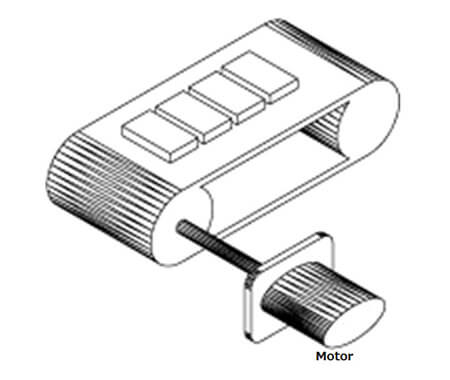最大容许转矩
• Pg = (P1 + P2 + P3) x 100 / η [W]
• P1 = 9.8 x μ x W x V x λ [W]
• P2 = (μ x Q x λ) / 367 [W]
• P3 = ± (Q x H) / 367 [W]
• λ：输送机之长 (m)
• W：皮带单位长之重量 (kg/m)
• μ：摩擦系数
• V：皮带速度 (m/sec)
• Q：输送量 (kg/h)
• η：效率 (%)
• H：倾斜输送机两端之高低差 (m)
将荷重卷上的场合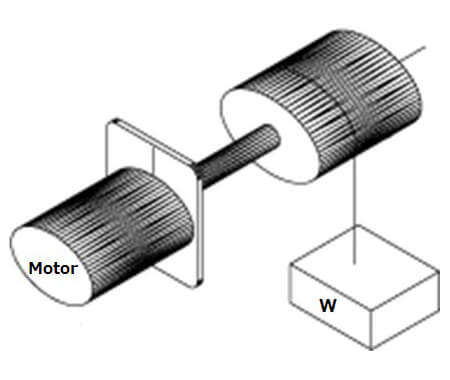Pg = (W x V) / (6 x 12) x (100/η) [W]

驱动惯性体的场合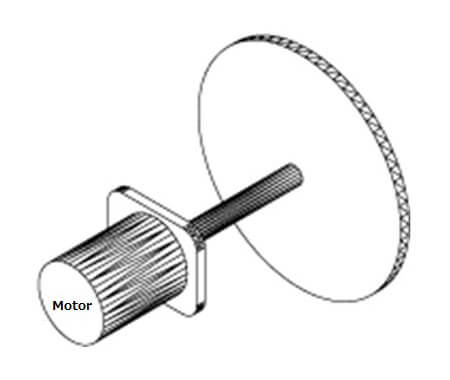• Pg = 1.027 NT [W]
• T ≒ (GD² /375) x (N/t) [kgf x m]
• N：每分钟回转数 (rpm)
• T：转矩 (kgf x m)
• GD²：飞轮效应 (kgf x m2) (含转子的飞轮效应)
• t：启动时间 (sec)
接触面上做水平移动的场合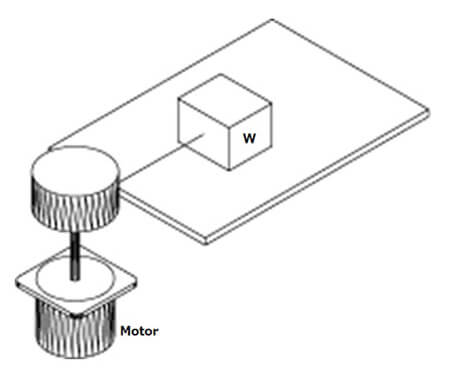Pg = (μ x W x V) / (6 x 12) [W]